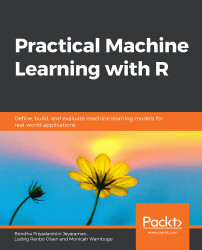•#### Practical Machine Learning with R#### Overview of this book

With huge amounts of data being generated every moment, businesses need applications that apply complex mathematical calculations to data repeatedly and at speed. With machine learning techniques and R, you can easily develop these kinds of applications in an efficient way. Practical Machine Learning with R begins by helping you grasp the basics of machine learning methods, while also highlighting how and why they work. You will understand how to get these algorithms to work in practice, rather than focusing on mathematical derivations. As you progress from one chapter to another, you will gain hands-on experience of building a machine learning solution in R. Next, using R packages such as rpart, random forest, and multiple imputation by chained equations (MICE), you will learn to implement algorithms including neural net classifier, decision trees, and linear and non-linear regression. As you progress through the book, you’ll delve into various machine learning techniques for both supervised and unsupervised learning approaches. In addition to this, you’ll gain insights into partitioning the datasets and mechanisms to evaluate the results from each model and be able to compare them. By the end of this book, you will have gained expertise in solving your business problems, starting by forming a good problem statement, selecting the most appropriate model to solve your problem, and then ensuring that you do not overtrain it.Free Chapter
An Introduction to Machine LearningData Cleaning and Pre-processingFeature EngineeringIntroduction to neuralnet and Evaluation MethodsLinear and Logistic Regression ModelsUnsupervised LearningAppendix## Introduction

Machine learning is a process through which we use data to train models. These models are then used to make predictions on a new set of data that the model hasn't seen before. There are different types of machine learning models, such as supervised learning, unsupervised learning, semi-supervised learning, and reinforcement learning.

The data in a supervised learning model should have labels or an end result. Supervised learning models are broadly classified into classification and regression learning models. In an unsupervised learning process, we may not know the labels or the outcomes beforehand. Clustering is an example of unsupervised learning. Semi-supervised learning models use a combination of supervised and unsupervised learning. In reinforcement learning, an agent learns to navigate an environment with feedback mechanisms that reinforce the goal maximizing actions.

In this chapter, the machine learning process will be demonstrated through examples. The types of machine learning models will be explained and the different evaluation metrics are discussed. We will learn to perform exploratory data analysis and implement a simple linear model in R.